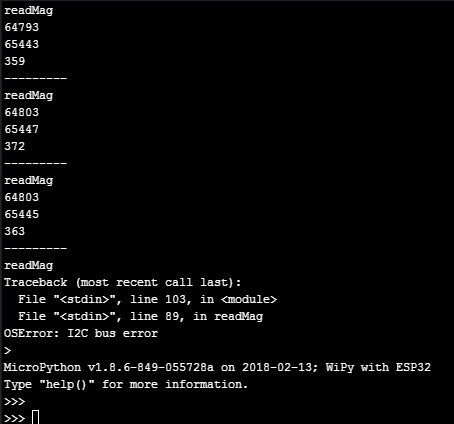# problems with I2C (OSError: I2C bus error)

• Hi";
i'm developing a driver for connecting the I2C based MAG3110 magnetometer to the Wipy, and i'm experiencing somre problems i don't know how to solve. the problem is that with the same code, sometimes it works ok, and i get the x, y and z values, and sometimes i get an "OSError: I2C bus error" message. where can be the problem? any help will be really appreciated. thanks

Note: No external pull-up resistors are used

``````from machine import I2C
import binascii
import time

_MAG3110_DR_STATUS = const(0x00)
_MAG3110_OUT_X_MSB = const(0x01)
_MAG3110_WHO_AM_I = const(0x07)
_MAG3110_CTRL_REG1 = const(0x10)
_MAG3110_CTRL_REG2 = const(0x11)
_MAG3110_WHO_AM_I_RSP = const(0xC4)
_MAG3110_ACTIVE_MODE = const(0x01)

_MAG3110_X_AXIS = const(1)
_MAG3110_Y_AXIS = const(3)
_MAG3110_Z_AXIS = const(5)

class MAG3110:
self.i2c = i2c
self.value = 0
self.reg = bytearray(1)
self.calibrationMode = False
self.activeMode = False
self.rawMode = False
self.calibrated = False

def setOffset(self, axis, offset):
print("setOffset")
offset = offset << 1
print((bytes((offset >> 8) & 0xFF)))
time.sleep_ms(15);

def reset(self):
print("reset")
self.calibrationMode = False
self.activeMode = False
self.rawMode = False
self.calibrated = False
self.setOffset(_MAG3110_X_AXIS, 0)
self.setOffset(_MAG3110_Y_AXIS, 0)
self.setOffset(_MAG3110_Z_AXIS, 0)

def initialize(self):
print("initialize")
print(binascii.hexlify(whoami))
if whoami == _MAG3110_WHO_AM_I_RSP:
print("MAG3110 connected");
self.reset()
return True
else:
print("Could not find MAG3110 connected!");
return False

def start(self):
print("start")
self.activeMode = True
self.reg |= _MAG3110_ACTIVE_MODE

return((self.reg & 0x08) >> 3)

data = bytearray(6)
x_t = (data << 8) + data
y_t = (data << 8) + data
z_t = (data << 8) + data
return x_t, y_t, z_t

i2c = I2C(0, I2C.MASTER, baudrate=400000)

mag3110_m.initialize()
mag3110_m.start()

while(True):
print(x)
print(y)
print(z)
print("---------")
time.sleep(5)
``````

• I'm testing this code with the correct frequency but i had probleme call "OSError: I2C bus error" in initialisation.
i use the sipy on the P9 and P10 and i didn't use int out
it's on this lines
print("initialize")
print(binascii.hexlify(whoami))

• Note: No external pull-up resistors are used

Any reason for no pull-ups?

• Hi @seb, yes, you are right ... i tried with 100k and it improves, but still appears some I2C errors. thanks

• @fbt

The datasheet for that sensor states that the maximum clock is 400KHz, you said you are using 4000000, which is 4MHz but looking at your code you have correctly set it to 400KHz. The datasheet says the clock can be between 0 and 400KHz so just to test it try lowering the clock down to 100KHz (standard speed) and see if this does improve anything

• @fbt Hia gain, attached you can find a sample output ... @jmarcelino , can it be related to the use of a baudrate of 4000000 ... any clue will be appreciated. thanksimage url)